×#### Thank you for registering.

One of our academic counsellors will contact you within 1 working day.

Click to Chat

1800-1023-196

+91-120-4616500

CART 0

• 0

MY CART (5)

Use Coupon: CART20 and get 20% off on all online Study Material

ITEM
DETAILS
MRP
DISCOUNT
FINAL PRICE
Total Price: Rs.

There are no items in this cart.
Continue Shopping• Complete JEE Main/Advanced Course and Test Series
• OFFERED PRICE: Rs. 15,900
• View Details

```Chapter 13: Linear Equation in Two Variables Exercise – 13.3

Question: 1

Draw the graph of each of the following linear equations in two variables:

(i) x + y = 4

(ii) x - y = 2

(iii) -x + y = 6

(iv) y = 2x

(v) 3x + 5y = 15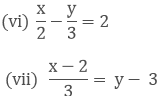(viii) 2y = -x +1

Solution:

(i) We are given, x + y = 4

We get, y = 4 - x,

Now, substituting x = 0 in y = 4 - x,

We get y = 4

Substituting x = 4 in y = 4 - x, we get y = 0

Thus, we have the following table exhibiting the abscissa and ordinates of points on the line represented by the given table

X
0
4

Y
4
0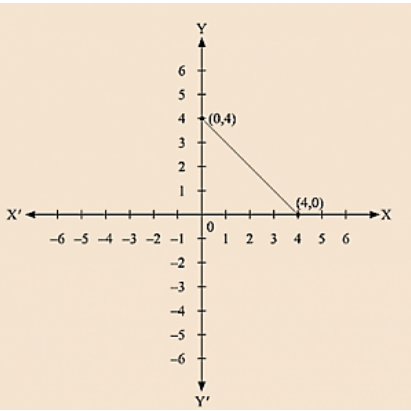(ii) We are given, x - y = 2

We get, y = x – 2

Now, substituting x = 0 in y= x – 2, we get y = - 2

Substituting x = 2 in y = x – 2, we get y = 0

Thus, we have the following table exhibiting the abscissa and ordinates of points on the line represented by the given equation

X
0
2

Y
- 2
0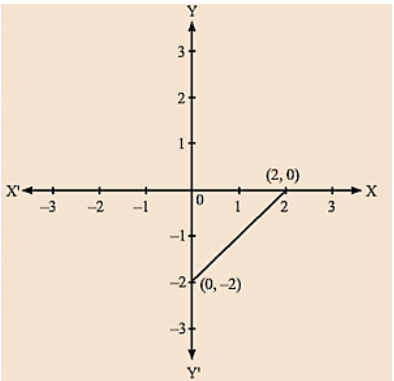(iii) We are given, - x + y = 6

We get, y = 6 + x

Now, substituting x = 0 in y = 6 + x,

We get y = 6

Substituting x = - 6 in y = 6 + x, we get y = 0

Thus, we have the following table exhibiting the abscissa and ordinates of points on the line represented by the given equation.

X
0
- 6

Y
6
0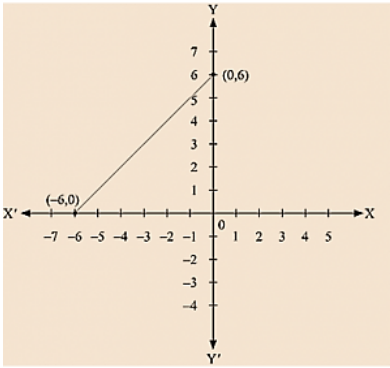(iv) We are given, y = 2x

Now, substituting x = 1 in y = 2x, we get y = 2

Substituting x = 3 in y = 2x,  we get y = 6

Thus, we have the following table exhibiting the abscissa and ordinates of points on the line represented by the given equation

X
1
3

Y
2
6(v) We are given, 3x + 5y = 15

We get, 15 - 3x = 5y

Now, substituting x = 0 in 5y = 15 - 3x,

We get; 5y = 15 y = 3

Substituting x = 5 in 5y = 15 – 3x we get 5y = 0

Thus, we have the following table exhibiting the abscissa and ordinates of points on the line represented by the given equation

X
0
5

Y
3
0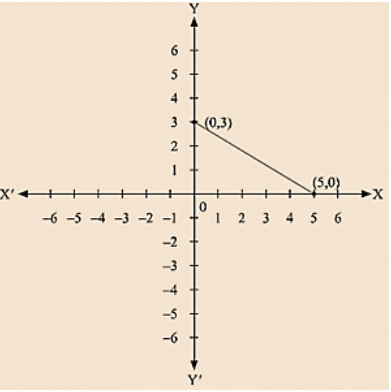(vi) we are given.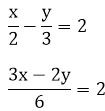3x - 2y = 12

We get,We get y = - 6

Substituting x = 4 inWe get y = 0 Thus, we have the following table exhibiting the abscissa and ordinates of points on the line represented by the given equation

X
0
4

Y
- 6
0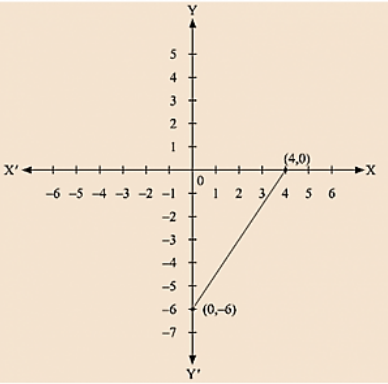(vii) We are given,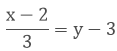We get, x - 2 = 3(y - 3)

x - 2 = 3y - 9

x = 3y - 7

Now, substituting x = 5 in x = 3y - 7,

We get; y = 4

Substituting x = 8 in x = 3y - 7,

We get; y = 5

Thus, we have the following table exhibiting the abscissa and ordinates of points on the line represented by the given equation

X
5
8

Y
4
5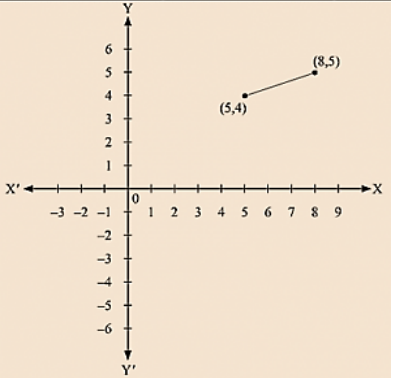(viii) We are given, 2y = - x +1

We get, 1 – x = 2y

Now, substituting x = 1 in 1– x = 2y, we get y = 0

Substituting x = 5 in 1 – x = 2y, we get y = - 2

Thus, we have the following table exhibiting the abscissa and ordinates of points on the line represented by the given equation

X
1
5

Y
0
- 2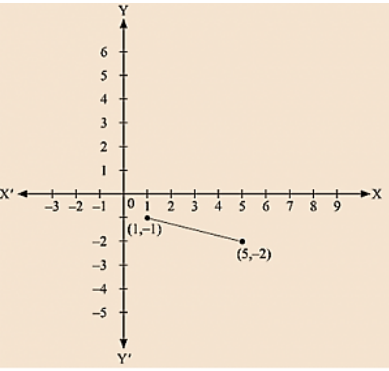Question: 2

Give the equations of two lines passing through (3, 12). How many more such lines are there, and why?

Solution:

We observe that x = 3 and y = 12 is the solution of the following equations 4x – y = 0 and 3x – y + 3 = 0

So, we get the equations of two lines passing through (3, 12) are, 4x - y = 0 and 3x - y + 3 = 0.

We know that passing through the given point infinitely many lines can be drawn. So, there are infinitely many lines passing through (3, 12)

Question: 3

A three-wheeler scooter charges Rs 15 for first kilometer and Rs 8 each for every subsequent kilometer. For a distance of x km, an amount of Rs y is paid. Write the linear equation representing the above information.

Solution:

Total fare of Rs y for covering the distance of x km is given by y = 15 + 8(x - 1)

y = 15 + 8x - 8

y = 8x + 7

Where, Rs y is the total fare (x - 1) is taken as the cost of first kilometer is already given Rs 15 and 1 has to subtracted from the total distance travelled to deduct the cost of first Kilometer.

Question: 4

A lending library has a fixed charge for the first three days and an additional charge for each day thereafter. Aarushi paid Rs 27 for a book kept for seven days. If fixed charges are Rs x and per day charges are Rs y. Write the linear equation representing the above information.

Solution:

Total charges of Rs 27 of which Rs x for first three days and Rs y per day for 4 more days is given by x + y (7 – 3) = 27

x + 4y = 27

Here, (7 - 3) is taken as the charges for the first three days are already given at Rs x and we have to find the charges for the remaining four days as the book is kept for the total of 7 days.

Question: 5

A number is 27 more than the number obtained by reversing its digits. lf its unit's and ten's digit are x and y respectively, write the linear equation representing the statement.

Solution:

The number given to us is in the form of ‘yx‘,

Where y represents the ten's place of the number And x represents the unit's place of the number.

Now, the given number is 10y + x

Number obtained by reversing the digits of the number is 10x + y It is given to us that the original number is 27 more than the number obtained by reversing its digits So, 10y + x = 10x + y + 27

10y – y + x - 10x = 27

9y - 9x = 27

9(y – x) = 27

y – x = 27/9

3x – y + 3 = 0

Question: 6

The Sum of a two digit number and the number obtained by reversing the order of its digits is 121. If units and tens digit of the number are x and y respectively, then write the linear equation representing the above statement.

Solution:

The number given to us is in the form of ‘yx’,

Where y represents the ten's place of the number and x represents the units place of the number

Now, the given number is 10y + x

Number obtained by reversing the digits of the number is 10x+ y

It is given to us that the sum of these two numbers is 121 So, (10y + x) + (10x + y) = 121

10y + y + x + 10x = 121

11y + 11x = 121

11(y + x) = 121

x + y = 121/11 = 11

x + y = 11

Question: 7

Plot the Points (3, 5) and (-1, 3) on a graph paper and verify that the straight line passing through the points, also passes through the point (1, 4)

Solution: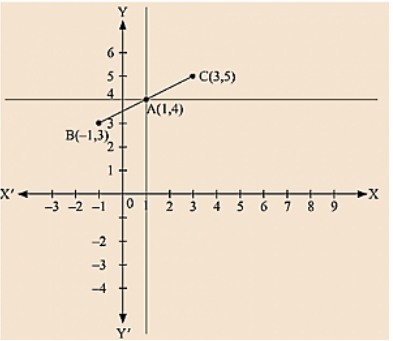By plotting the given points (3, 5) and (-1, 3) on a graph paper, we get the line BC.

We have already plotted the point A (1, 4) on the given plane by the intersecting lines.

Therefore, it is proved that the straight line passing through (3, 5) and (-1, 3) also passes through A (1, 4).

Question: 8

From the choices given below, choose the equations whose graph is given in fig

(i) y = x

(ii) x + y = 0

(iii) y = 2x

(iv) 2 + 3y = 7x

Solution:

We are given co-ordinates (1, - 1) and (-1, 1) as the solution of one of the following equations.

We will substitute the value of both co-ordinates in each of the equation and find the equation which satisfies the given co-ordinates.(i) We are given, y = x

Substituting x =I and y = - 1, we get; 1 ≠ -1

L.H.S ≠ R.H.S

Substituting x = -1 and y = 1, we get; – 1 ≠1

L.H.S ≠ R.H.S

Therefore, the given equation y = x does not represent the graph in the figure.

(ii) We are given, x + y = 0

Substituting x = 1 and y = -1, we get

⟹ 1 + (-1) = 0

⟹ 0 = 0

L.H.S = R.H.S

Substituting x = 1 and y = 1, we get (-1) + 1 = 0

0 = 0

L.H.S = R.H.S

Therefore, the given solutions satisfy this equation.

Thus, it is the equation whose graph is given.

Question: 9

From the choices given below, choose the equation whose graph is given fig:

(i) y = x + 2

(ii) y = x – 2

(iii) y = - x + 2

(iv) x + 2y = 6

Solution:

We are given co-ordinates (-1, 3) and (2, 0) as the solution of one of the following equations.

We will substitute the value of both co-ordinates in each of the equation and find the equation which satisfies the given co-ordinates.

(i) We are given, y=x+2 Substituting x = - 1 and y = 3,We get 3 ≠ -1+ 2

L.H.S ≠ R.H.S

Substituting x = 2 and y = 0,

We get 0 ≠ 4

L.H.S ≠ R.H.S

Therefore, the given solution does not satisfy this equation.

(ii) We are given, y = x - 2

Substituting x = 1 and y = 3,

We get 3 = - 1 - 2

L.H.S ≠ R.H.S

Substituting x = 2 and y = 0, we get 0 = 0

L.H.S = R.H.S

Therefore, the given solutions does not completely satisfy this equation.

(iii) We are given, y = - x + 2

Substituting x = - 1 and y = 3,

We get 3 = - (-1) + 2

L.H.S = R.H.S

Substituting x = 2 and y = 0, we get 0 = - 2 + 2

0 = 0

L.H.S = R.H.S

Therefore, the given solutions satisfy this equation. Thus, it is the equation whose graph is given.

Question: 10

If the point (2, -2) lies on the graph of linear equation, 5x + 4y = 4, find the value of k.

Solution:

It is given that the point (2,-2) lies on the given equation, 5x + ky = 4

Clearly, the given point is the solution of the given equation.

Now, Substituting x = 2 and y = - 2 in the given equation, we get 5x + ky = 4

5 × 2 + (- 2) k = 4

2k = 10 – 4

2k = 6

k = 6/2

k = 3

Question: 11

Draw the graph of equation 2x + 3y = 12.

From the graph, find the co-ordinates of the point:

(i) whose y-coordinate is 3

(ii) whose x coordinate is -3

Solution:

We are given, 2x + 3y = 12

We get,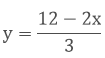Substituting, x = 0 inWe get,y = 4

Substituting x = 6 in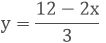y = 12 – 2 × 63

y = 0

Thus, we have the following table exhibiting the abscissa and ordinates of points on the line represented by the given equation

X
0
6

Y
4
0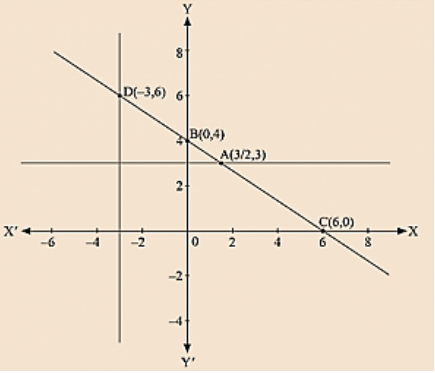By plotting the given equation on the graph, we get the point B(0, 4) and C (6,0).

(i) Co-ordinates of the point whose y axis is 3 are A (3/2, 3)

(ii) Co-ordinates of the point whose x -coordinate is -3 are D (- 3, 6)

Question: 12

Draw the graph of each of the equations given below. Also, find the coordinates of the points where the graph cuts the coordinate axes:

(i) 6x – 3y = 12

(ii) - x + 4y = 8

(iii) 2x + y = 6

(iv) 3x + 2y + 6 = 0

Solution:

(i) We are given, 6x - 3y = 12

We get, y = (6x -12)/3

Now, substituting x = 0 in y = - (6x – 12)/3

we get y = - 4

Substituting x = 2 in y = (- 6x -12)/3, we get y = 0

Thus, we have the following table exhibiting the abscissa and ordinates of points on the line represented by the given equation

X
0
2

Y
- 4
0Co-ordinates of the points where graph cuts the co-ordinate axes are y = - 4 at y axis and x = 2 at x axis.

(ii) We are given, - x + 4y = 8

We get,Now, substituting x = 0 inwe get y = 2

Substituting x = - 8 inWe get y = 0

Thus, we have the following table exhibiting the abscissa and ordinates of points on the line represented by the given equation

X
0
- 8

Y
2
0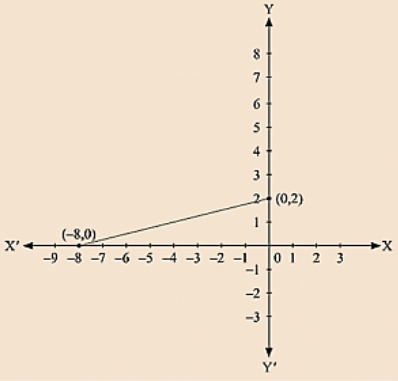Co-ordinates of the points where graph cuts the co-ordinate axes are y = 2 at y axis and x = -8 at x axis.

(iii) We are given, 2x + y = 6

We get, y = 6 - 2x

Now, substituting x = 0 in y = 6 - 2x we get y = 6

Substituting x = 3 in y = 6 - 2x, we get y = 0

Thus, we have the following table exhibiting the abscissa and ordinates of points on the line represented by the given equation

X
0
3

Y
6
0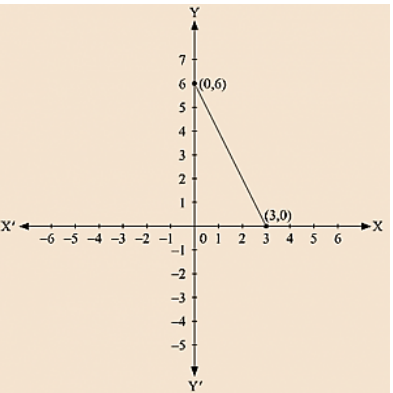Co-ordinates of the points where graph cuts the co-ordinate axes are y = 6 at y axis and x = 3 at x axis.

(iv) We are given, 3x + 2y + 6 = 0

We get,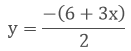Now, substituting x = 0 inSubstituting x = 2 inwe get y = 0

Thus, we have the following table exhibiting the abscissa and ordinates of points on the line represented by the given equation

X
0
- 2

Y
- 3
0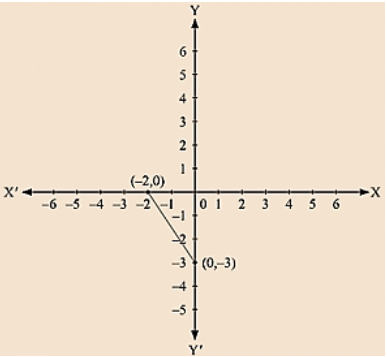Co-ordinates of the points where graph cuts the co-ordinate axes are y = - 3 at y axis and x = - 2 at x axis.

Question: 13

Draw the graph of the equation 2x + y = 6. Shade the region bounded by the graph and the coordinate axes. Also, find the area of the shaded region.

Solution:

We are given, 2x + y = 6

We get, y = 6 - 2x

Now, substituting x = 0 in y = 6 - 2x,

we get y = 6

Substituting x = 3 in y = 6 - 2x, we get y = 0

Thus, we have the following table exhibiting the abscissa and ordinates of points on the line represented by the given equation

X
0
3

Y
6
0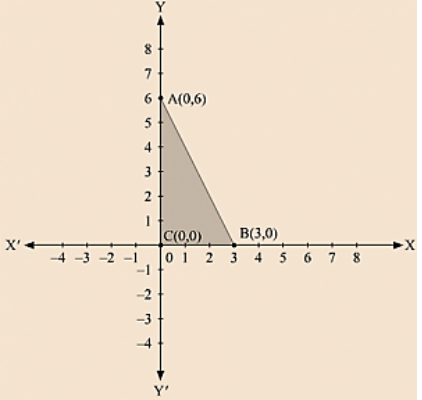The region bounded by the graph is ABC which forms a triangle.

AC at y axis is the base of triangle having AC = 6 units on y axis.

BC at x axis is the height of triangle having BC = 3 units on x axis.

Therefore, Area of triangle ABC, say A is given by A = (Base x Height)/2

A = (AC × BC)/2

A = (6 × 3)/2

A = 9 sq. units

Question: 14

Draw the graph of the equation x/3 + y/4 = 1. Also, find the area of the triangle formed by 3/4 the line and the coordinates axes.

Solution:

We are given. x/3 + y/4 = 1

4x + 3y =12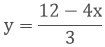Now, substituting x = 0 inwe get y = 4

Substituting x = 3 inwe get y = 0

Thus, we have the following table exhibiting the abscissa and ordinates of points on the line represented by the given equation

X
0
3

Y
4
0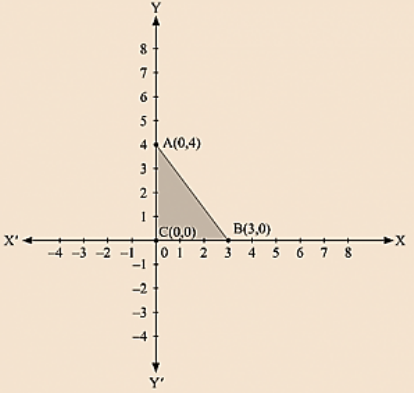The region bounded by the graph is ABC which forms a triangle.

AC at y axis is the base of triangle having AC = 4 units on y axis.

BC at x axis is the height of triangle having BC = 3 units on x axis.

Therefore, Area of triangle ABC, say A is given by A = (Base × Height)/2

A= (AC × BC)/2

A = (4 × 3)/2

A = 6 sq. units

Question: 15

Draw the graph of y = |×|.

Solution:

We are given, y = |x|

Substituting x = 1, we get y = 1

Substituting x = - 1, we get y = 1

Substituting x = 2, we get y = 2

Substituting x = - 2, we get y = 2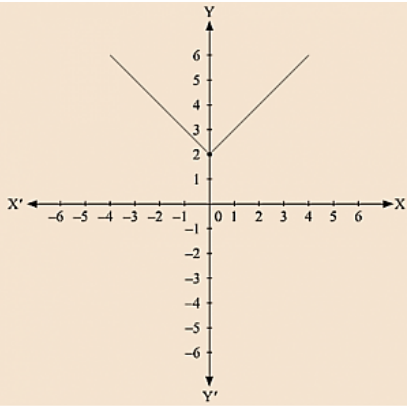For every value of x, whether positive or negative, we get y as a positive number.

Question: 16

Draw the graph of y = |x| + 2.

Solution:

We are given, y = |x| + 2

Substituting x = 0 we get y = 2

Substituting x = 1, we get y = 3

Substituting x = - 1, we get y = 3

Substituting x = 2, we get y = 4

Substituting x = - 2, we get y = 4For every value of x, whether positive or negative, we get y as a positive number and the minimum value of y is equal to 2 units.

Question: 17

Draw the graphs of the following linear equations on the same graph paper: 2x + 3y = 12, x - y = 1 Find the coordinates of the vertices of the triangle formed by the two straight lines and the y-axis. Also, find the area of the triangle.

Solution:

We are given, 2x +3y =12

We get,Now, substituting x = 0 inwe get y = 4

Substituting x = 6 inwe get y = 0

Thus, we have the following table exhibiting the abscissa and ordinates of points on the line represented by the given equation

X
0
6

Y
4
0

Plotting A (0, 4) and E(6, 0) on the graph and by joining the points ,

we obtain the graph of equation 2x + 3y = 12.

We are given, x – y = 1

We get, y = x – 1

Now, substituting x = 0 in y = x - 1, we get y = -1

Substituting x in y = x - 1, we get y = -2

Thus, we have the following table exhibiting the abscissa and ordinates of points on the line represented by the given equation

X
0
-1

Y
-1
-2

Plotting D (0, – 1) and E (-1, 0) on the graph and by joining the points, we obtain the graph of equation x - y = 1.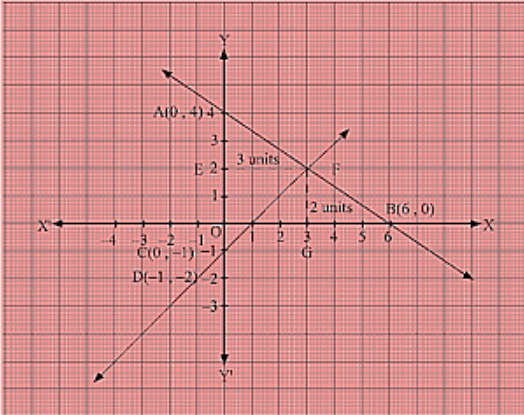By the intersection of lines formed by 2x + 3y = 12 and x - y = 1 on the graph, triangle ABC is formed on y axis.

Therefore, AC at y axis is the base of triangle ABC having AC = 5 units on y axis.

Draw FE perpendicular from F on y axis.

FE parallel to x axis is the height of triangle ABC having FE = 3 units on x axis.

Therefore, Area of triangle ABC, say A is given by A = (Base × Height)/2 = (AC × FE)/2 = (5× 3)/2 =>15/2 = 7.5 sq. units

Question: 18

Draw the graphs of the linear equations 4x - 3y + 4 = 0 and 4x + 3y - 20 = 0. Find the area bounded by these lines and x-axis.

Solution:

We are given, 4x - 3y + 4 = 0

We get,Now, substituting x = 0 inwe get

Substituting x = -1 inwe get y = 0

Thus, we have the following table exhibiting the abscissa and ordinates of points on the line represented by the given equation

x
0
-1

y
3/4
0

Plotting E(0, 4/3) and A (-1, 0) on the graph and by joining the points,

We obtain the graph of equation 4x - 3y + 4 = 0.

We are given, 4x + 3y - 20 = 0

We get,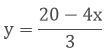Now, substituting x = 0 inwe get y = 7

Substituting x = 5 inwe get y = 0

Thus, we have the following table exhibiting the abscissa and ordinates of points on the line represented by the given equation

X
0
5

Y
20/3
0

Plotting D (0, 20/3) and B (5, 0) on the graph and by joining the points, we obtain the graph of equation 4x +3y - 20 = 0.By the intersection of lines formed by 4x - 3y + 4 = 0 and 4x + 3y - 20 = 0 on the graph,

Triangle ABC is formed on x axis.

Therefore, AB at x axis is the base of triangle ABC having AB = 6 units on x axis.

Draw CF perpendicular from C on x axis. CF parallel to y axis is the height of triangle ABC having CF = 4 units on y axis.

Therefore, Area of triangle ABC, say A is given by A = (Base × Height)/2

A = (AB × CF)/2

A = (6 × 4)/2

K = 12 sq. units

Question: 19

The path of a train A is given by the equation 3x + 4y -12 = 0 and the path of another train B is given by the equation 6x + 8y - 48 = 0. Represent this situation graphically.

Solution:

We are given the path of train A, 3x + 4y - 12 = 0

We get,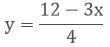Now, substituting x = 0 inwe get y = 3

Substituting x = 4 inwe get y = 0

Thus, we have the following table exhibiting the abscissa and ordinates of points on the line represented by the given equation

X
0
4

Y
3
0

Plotting A(4, 0) and E(0, 3) on the graph and by joining the points,

we obtain the graph of equation 3x + 4y - 12 = 0.

We are given the path of train B, 6x + 8y - 48 = 0

We get,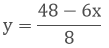Now, substituting x = 0 inwe get y = 6

Substituting x = 8 inwe get y = 0

Thus, we have the following table exhibiting the abscissa and ordinates of points on the line represented by the given equation

X
0
8

Y
6
0

Plotting C (0, 6) and D (8, 0) on the graph and by joining the points. We obtain the graph of equation 6x + 8y – 48 = 0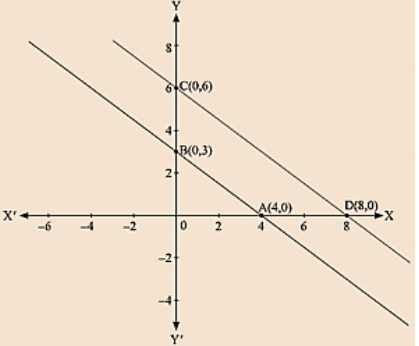Question: 20

Ravish tells his daughter Aarushi, "Seven years ago, I was seven times as old as you were then. Also, three years from now, I shall be three times as old as you will be". If present ages of Aarushi and Ravish are x and y years respectively, represent this situation algebraically as well as graphically.

Solution:

We are given the present age of Ravish as y years and Aarushi as x years.

Age of Ravish seven years ago = y - 7

Age of Aarushi seven years ago = x – 7

It has already been said by Ravish that seven years ago he was seven times old then Aarushi was then So, y - 7 = 7( x – 7)

y – 7 = 7x – 49

7x - y = - 7 + 49

7x – y – 42 = 0 .... (1)

Age of Ravish three years from now = y + 3

Age of Aarushi three years from now = x + 3

It has already been said by Ravish that three years from now he will be three times older then Aarushi will be then So, Y + 3 = 3 (x + 3)

y + 3 = 3x + 9

3x + 9 – y – 3 = 0

3x – y + 6 = 0 ..... (2)

(1) and (2) are the algebraic representation of the given statement.

We are given, 7x - y- 42 = 0

We get, y = 7x – 42

Now, substituting x = 0 in y = 7x – 42, we get y = – 42

Substituting x = 6 in y = 7x - 42, we get y = 0

Thus, we have the following table exhibiting the abscissa and ordinates of points on the line represented by the given equation

X
0
6

Y
- 42
0

We are given, 3x – y + 6 = 0

We get, Y = 3x + 6

Now, substituting x = 0 in y = 3x + 6, We get y = 6

Substituting x = -2 in y = 3x + 6,

We get y = 0

Thus, we have the following table exhibiting the abscissa and ordinates of points on the line represented by the given equation

X
0
-2

Y
6
0The red – line represents the equation 7x - y - 42 = 0.

The blue-line represents the equation 3x - y + 6 = 0.

Question: 21

Aarushi was driving a car with uniform speed of 60 km/h. Draw distance-time graph From the graph, find the distance travelled by Aarushi in

(i) 5/2 Hours

(ii) 1/2 Hour

Solution:

Aarushi is driving the car with the uniform speed of 60 km/h. We represent time on X-axis and distance on Y-axis Now, graphically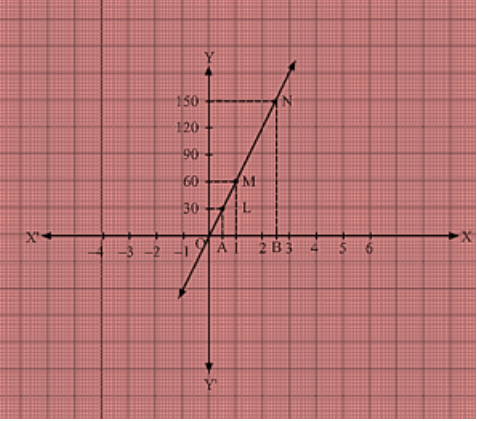We are given that the car is travelling with a uniform speed 60 km/hr.

This means car travels 60 km distance each hour.

Thus the graph we get is of a straight line.

Also, we know when the car is at rest, the distance travelled is 0 km, speed is 0 km/hr and the time is also 0 hr.

Thus, the given straight line will pass through O(0, 0) and M(1, 60).

Join the points 0 and M and extend the line in both directions.

Now, we draw a dotted line parallel to y-axis from x = 12 that meets the straight line graph at L from which we draw a line parallel to x-axis that crosses y-axis at 30.

Thus, in 12 hr, distance travelled by the car is 30 km.

Now, we draw a dotted line parallel to y-axis from x = 5/2  that meets the straight line graph at N from which we draw a line parallel to x-axis that crosses y-axis at 150.

Thus, in 5/2 hr, distance travelled by the car is 150 km.

(i) Distance = Speed × Time Distance travelled in 5/2 hours is given by

Distance = 60 × 5/2, Distance = 150 Km

(ii) Distance = Speed × Time Distance travelled in 12 hour is given by Distance = 60 × 5/2

Distance = 30 km
```### Course Features

• 728 Video Lectures
• Revision Notes
• Previous Year Papers
• Mind Map
• Study Planner
• NCERT Solutions
• Discussion Forum
• Test paper with Video Solution